# Paper 2 skills

## Map Skills

Q1 in Paper 2 is always map skills.  As long as you remember the basic techniques of map-work you should have no problem with this section.

### Grid References

Remember: go across first, then go up the side.

####4 Figure Grid References

4 figure grid references locate the square that a feature is in.

1. Orange circle =  01 10
2. Blue square = 02 11
3. Green Triangle =03 10

#### 6 Figure Grid References

These locate the exact position on a map.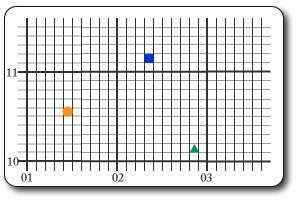Each grid square is broken into 100 smaller squares.

Find the grid line going across and the divide the square into tenths (like a decimal).

Do the same on the vertical axis.

Think of the extra digits as decimals but without the dot.

1. Blue square = 023 111  (the grid square is 02 11)
2. Orange Square = 014 105
3. Green triangle = 028 101

#### Measuring Height

The main way that height is shown on OS maps (used in the exam) is contour lines.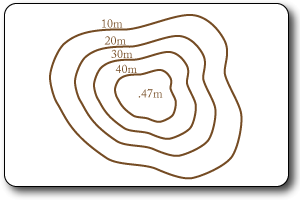These are brown lines that run along places that are the same height.

The closer together they are the steeper the slope.

Contour lines can vary in the height increase that they show (usually 10m or 20m) so check the map key.

Spot heights are used to show the top of a hill/mountain and they give the exact height in meters.

#### Measuring Distance

Straight line distance: measure the direct distance between the two stated points.  Use the scale underneath the map extract to work out the distance in km or miles.

Curved line distance: if asked to measure the distance along a road you need to use a piece of paper to measure the distance.  Mark the end of each straight section and the turn the paper following the curve to measure the next straight section.  Use the scale to give the correct distance.

Divide the horizontal distance by the vertical distance (500/100= 5) then display the answer as: 1 in 5

#### Using the Key

Remember that there will always be a key for the map that tells you what the symbols and colours mean.

You must use the key & pay attention to details (embankments vs cuttings).

State which type of road (Main A, Main B etc).

#### Practise Questions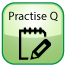## Photograph Interpretation & Analysis

There is always one question that requires you to identify and describe geographical/human features or evidence of processes.

To score well in this section you need to use geographic terms accurately in your description and where possible identify whether features are in the foreground or the background of the photograph.

If asked to describe relief terms use terms such as: steeply sloped, cliff, flat land, v-shaped valley, mountain.

If asked to describe the characteristics of a river use terms such as: meander, river bank, gorge, v-shaped, rapids, angular bedload, rocky, deep/shallow, wide/narrow.

If asked to describe vegetation use terms such as: sparse/dense, shrubs, cacti, abundant, trees, grasses, flowers.

## Graphs & Data

You are expected to be able to read data from a variety of different graph types and possibly complete unfinished graphs.

Make sure you state the unit of measurement when reading data from graphs (miles, meters, \$s etc).

You should also be able to suggest suitable graph types for any given data.

#### Compound Bar Charts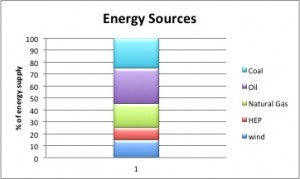These display data in a single bar.

The data may represent a % in which case the total will always be 100.

#### Bar & Column Charts

Bar charts are used to compare separate sets of data.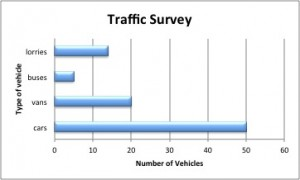Bar charts have horizontal lines

Columns charts have vertical lines.

Several sets of data may be included on one graph (traffic survey results conducted at different locations or times).

#### Line Graphs

line charts are used to show data over time to identify trends/patterns.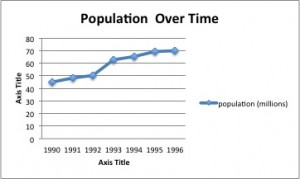They are used with continuous data such as population growth & GDP over time.

Scatter Graphs

These are used to show relationships between 2 sets of data.